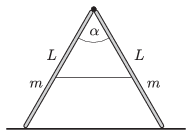Mathematical and Physical Journal
for High Schools
Issued by the MATFUND Foundation
 Already signed up? New to KöMaL?

#Problem P. 4631. (April 2014)

P. 4631. A step ladder is placed to the smooth horizontal surface of ice. The cross section of the ladder is the same everywhere and the angle between its legs is $\displaystyle \alpha=60^\circ$. The hinge which connects the two legs of mass 5 kg and of length of 2 m is frictionless. The thin, initially tight safety rope breaks.$\displaystyle a)$ What is the angle between the legs of the ladder, when the hinge does not exert any force?

$\displaystyle b)$ What is the force exerted on the ice by the ladder at this moment?

$\displaystyle c)$ At what speed does the hinge hit the ice?

(5 pont)

Deadline expired on May 12, 2014.

Sorry, the solution is available only in Hungarian. Google translation

Megoldásvázlat. $\displaystyle a)$ $\displaystyle 2\,{\rm arccos}\left(\frac23 \cos\frac{\alpha}{2}\right)\approx 109{,}5^\circ$.

$\displaystyle b)$ $\displaystyle F= mg/2=24{,}5~$N.

$\displaystyle c)$ $\displaystyle v=\sqrt{\frac{3\sqrt{3}}{2}gL}=7{,}14~$m/s.

### Statistics:

 27 students sent a solution. 5 points: Antalicz Balázs, Asztalos Bogdán, Balogh Menyhért, Csathó Botond, Forrai Botond, Horicsányi Attila, Juhász Péter, Kovács Péter Tamás, Sal Kristóf. 4 points: Berta Dénes, Blum Balázs, Bugár 123 Dávid, Dinev Georgi, Elahe Ahmadi, Fehér Zsombor, Fekete Panna, Holczer András, Janzer Barnabás, Marosvári Kristóf, Németh Flóra Boróka, Olosz Balázs, Sárvári Péter. 3 points: 1 student. 2 points: 2 students. 1 point: 1 student. 0 point: 1 student.

Problems in Physics of KöMaL, April 2014